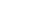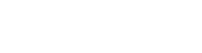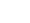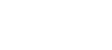# Soil Moisture Content-Dry Density RelationshipReading time: 1 minute

In compaction of soils, the main aim is to keep the soil particles close together which leads to improve dry density of soil. The soil with maximum dry density is suitable for the several constructional purposes. But maximum dry density of soil through compaction will be possible at a particular moisture content called optimum moisture content. Hence compaction purely depends upon the relationship between moisture content of soil and its dry density which is briefly discussed below.## Moisture content – dry density relationship

Moisture content of soil is defined as the ratio of mass of water to the mass of solids present in the soil sample. It is represented by “w”.Dry density of soil is defined as the ratio of Mass of solids to the total volume of the soil. It is represented byTo obtain relationship between the moisture content and dry density, multiply the numerator and denominator of expression of dry density with “M” which is mass of soil sample.Mass of the soil (M) is nothing but the addition of Mass of solids (Ms) and Mass of water (Mw).Ratio of mass to the volume is bulk density of soil which is denoted asTherefore,Hence to obtain dry density, we need bulk density and moisture content of soil sample.

## How moisture content affects the dry density?

Generally dry soil contains soil particles which are not in contact with each other and when we try to compact this type of soil without water tit becomes stiff and cracks and gaps will be formed. When we added water to it, the water forms a thin film around the each soil particle and this film helps the particles to contact with each other. Thereby the soil becomes denser under compaction. At one point of time, the volume of air in the soil sample becomes minimum and dry density of soil becomes maximum. This is called maximum dry density point at this point the addition of water should be stopped. The moisture content corresponding to the maximum dry density of soil is called as “Optimum moisture content”. If water is added beyond the optimum moisture content, the water will occupy the extra space since there is no air volume and dry density will reduce. This change in dry density values with respect to water content is shown by the compaction curve below.Compaction Curve

Compaction curve is plotted by taking moisture content on x-axis and dry density on y-axis. Required amount of soil is collected from the field and compaction test performed in the laboratory. The results obtained are used to draw the compaction curve as a result the amount of compaction required will be known. The laboratory result is approximately equal to the amount of compaction required in the field.